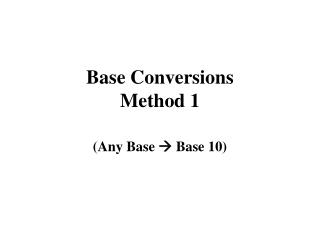DownloadDownload PresentationBase Conversions Method 1

# Base Conversions Method 1

Download Presentation## Base Conversions Method 1

- - - - - - - - - - - - - - - - - - - - - - - - - - - E N D - - - - - - - - - - - - - - - - - - - - - - - - - - -
##### Presentation Transcript

1. Base ConversionsMethod 1 (Any Base  Base 10)

2. e.g. 1024 is valid since each digit is less than the base. But 1535 is invalid; 5 is not less than the base.

3. The person who started with this number was most likely checking work done with method 2, since method 1 is used to check method 2.

4. 2) Determine the positional value for each digit. Starting with the rightmost position, give it a value of 1 (base to the zero power).

5. To determine the preceding positional values, multiply the current positional value by the base e.g. 3859 3 8 5 base 9 | | | 81 9 1

6. 3) Then multiply each digit by its positional valuee.g. 3 x 81 = 243 8 x 9 = 72 5 x 1 = 5

7. 3 x 81 = 243 8 x 9 = 72 5 x 1 = 54) and add them together ==== 320 this is the answer in base 10

8. 5) Finally, check the result for validity; each digit should be less than 10. (If it is not, then you did something wrong and should start over)